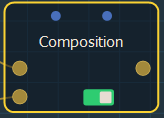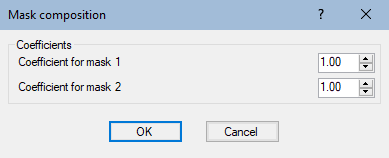This node creates a linear composition between two masks. At each vertex, the value of the output mask is equal to the value of the first input mask multiplied by the first coefficient, plus the value of the second input mask multiplied by the second coefficient. The second input mask is scaled to fit the size of the first input mask.

To add a node, right click in the Graph Editor and select Create Node  > Mask Composition > Composition.Double click on the node to open its parameters:Setting the coefficients

Set the coefficients for the first and second mask in the parameters dialog.

• Coefficient for the first terrain: The coefficient by which the height of the first input terrain is multiplied.
• Coefficient for the second terrain: The coefficient by which the height of the second input terrain is multiplied.

When both coefficients are equal to 1, the result is identical to that produced by the Sum node. When the first coefficient is equal to 1 and the second is equal to -1, the result is identical to that produced by the Difference node. When both coefficients are equal to 0.5, the result is identical to that produced by the Average node.

Parameters

Parameter Use
Coefficient for the first mask The coefficient by which the height of the first input mask is multiplied
Coefficient for the second mask The coefficient by which the height of the second input mask is multiplied